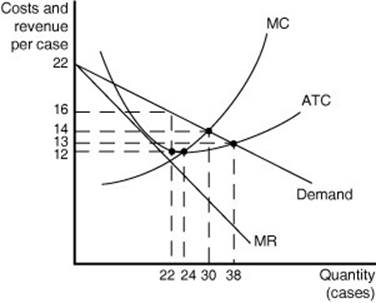### Create an Account

Home / Questions / The below Figure shows cost and demand curves for a monopolistically competitive producer ...

# The below Figure shows cost and demand curves for a monopolistically competitive producer of iced-tea Use the Figure to answer question 15 through 18 What is the profit-maximizing output level

The below Figure shows cost and demand curves for a monopolistically competitive producer of iced-tea. Use the Figure to answer question 15 through 18What is the profit-maximizing output level?

 A.22 cases B. 24 cases C. 30 cases D. 38 cases

What is the firm's profit-maximizing price?

 A 12 B 13 C 14 D 16

At the profit-maximizing output level the firm will

A. earn a profit of \$176.

B. break even.

C. earn a profit of \$88.

D. earn a profit of \$60

Based on the diagram, one can conclude that

 A. some existing firms will exit the market. B. new firms will enter the market. C. the industry is in long-run equilibrium. D. firms achieve productive efficiency

Apr 29 2020 View more View LessSubscribe To Get Solution